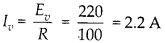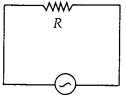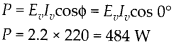Enlightened

# Question 1: NCERT Solutions for 12th Class Physics: Chapter 7-Alternating Current

• 0

Question 1: NCERT Solutions for 12th Class Physics: Chapter 7-Alternating Current

A 100 Q resistor is connected to a 220 V, 50 Hz a.c. supply.
(a) What is the rms value of current in the circuit?
(b) What is the net power consumed over a full cycle?

Share

1. Solution:
(a) Here virtual a.c. voltage is 220 V at a frequency of 50 Hz. So, rms value of current(b) Power in complete cycleCheck the complete chapter with solutions.

NCERT Solutions for 12th Class Physics: Chapter 7-Alternating Current

• 0